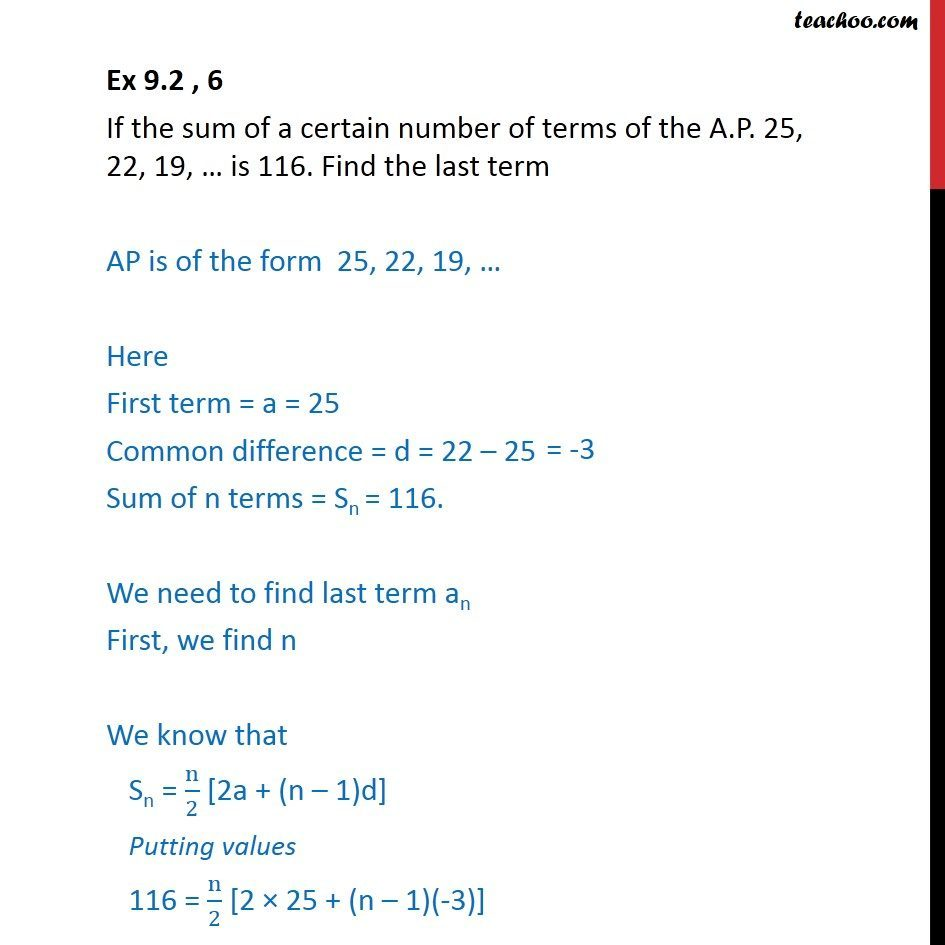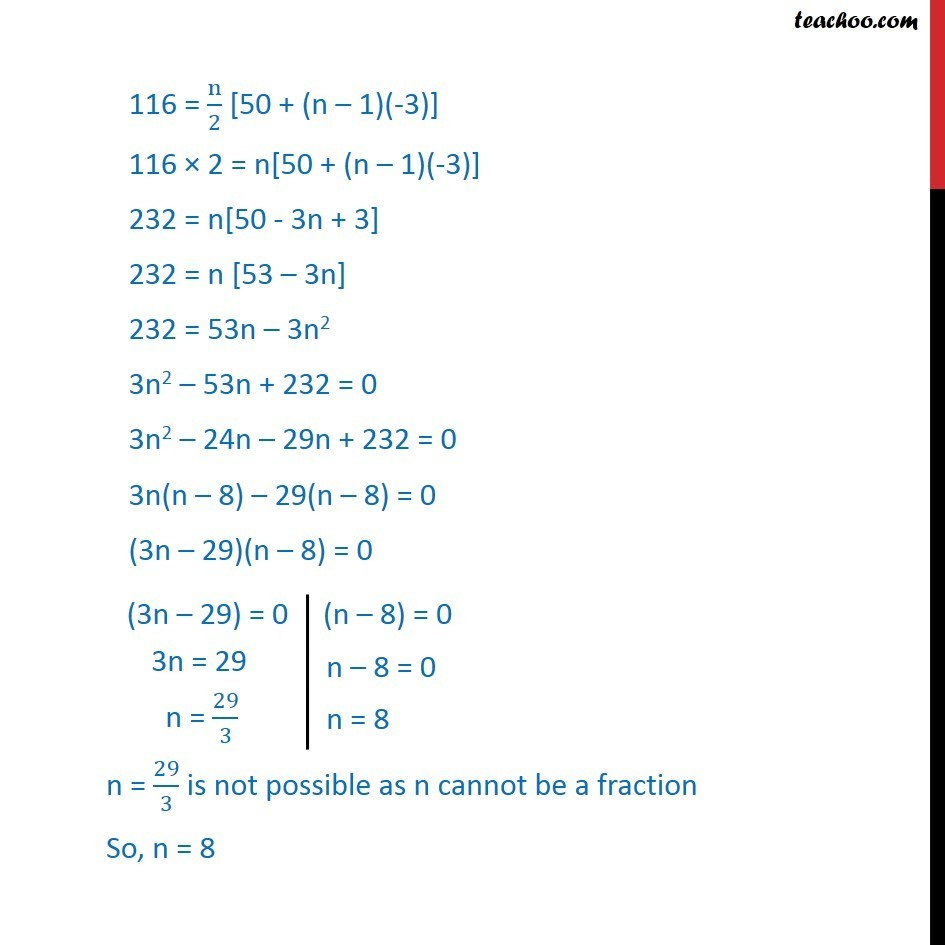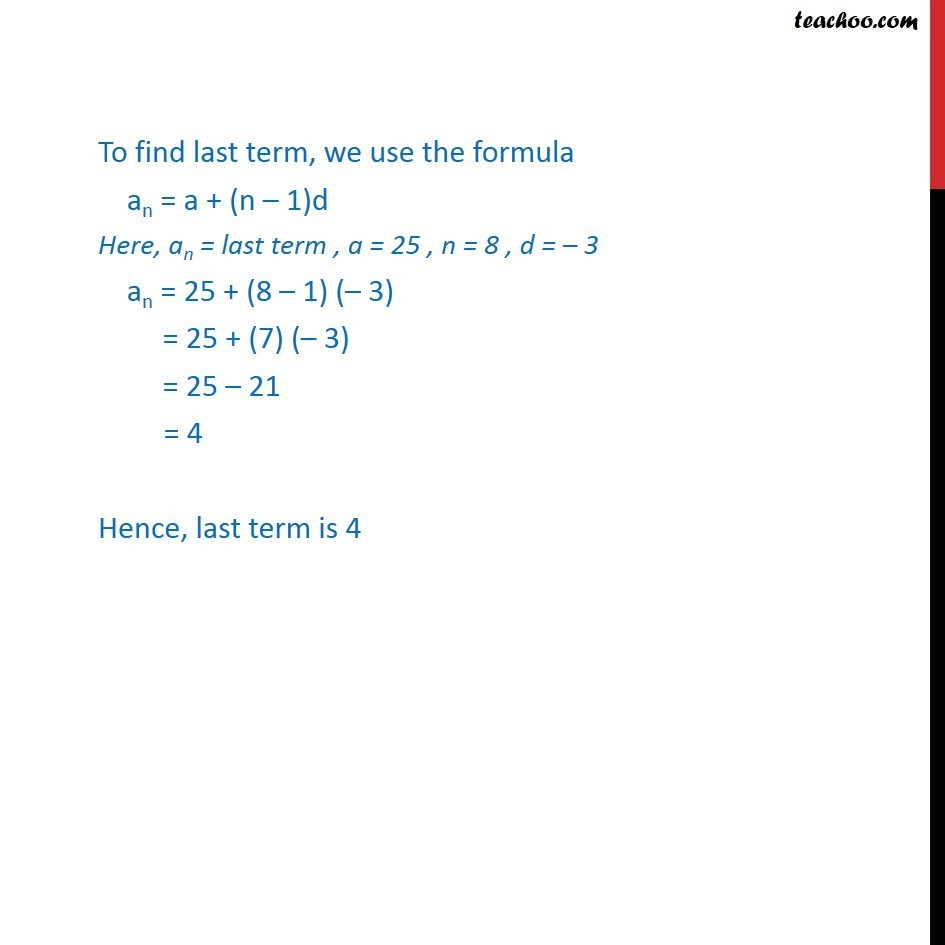Arithmetic Progression

Chapter 8 Class 11 Sequences and Series
Serial order wiseLearn in your speed, with individual attention - Teachoo Maths 1-on-1 Class

### Transcript

Ex 9.2 , 6 If the sum of a certain number of terms of the A.P. 25, 22, 19, … is 116. Find the last term AP is of the form 25, 22, 19, … Here First term = a = 25 Common difference = d = 22 – 25 Sum of n terms = Sn = 116. We need to find last term an First, we find n We know that Sn = n/2 [2a + (n – 1)d] Putting values 116 = n/2 [2 × 25 + (n – 1)(-3)] 116 = n/2 [50 + (n – 1)(-3)] 116 × 2 = n[50 + (n – 1)(-3)] 232 = n[50 - 3n + 3] 232 = n [53 – 3n] 232 = 53n – 3n2 3n2 – 53n + 232 = 0 3n2 – 24n – 29n + 232 = 0 3n(n – 8) – 29(n – 8) = 0 (3n – 29)(n – 8) = 0 n = 29/3 is not possible as n cannot be a fraction So, n = 8 To find last term, we use the formula an = a + (n – 1)d Here, an = last term , a = 25 , n = 8 , d = – 3 an = 25 + (8 – 1) (– 3) = 25 + (7) (– 3) = 25 – 21 = 4 Hence, last term is 4